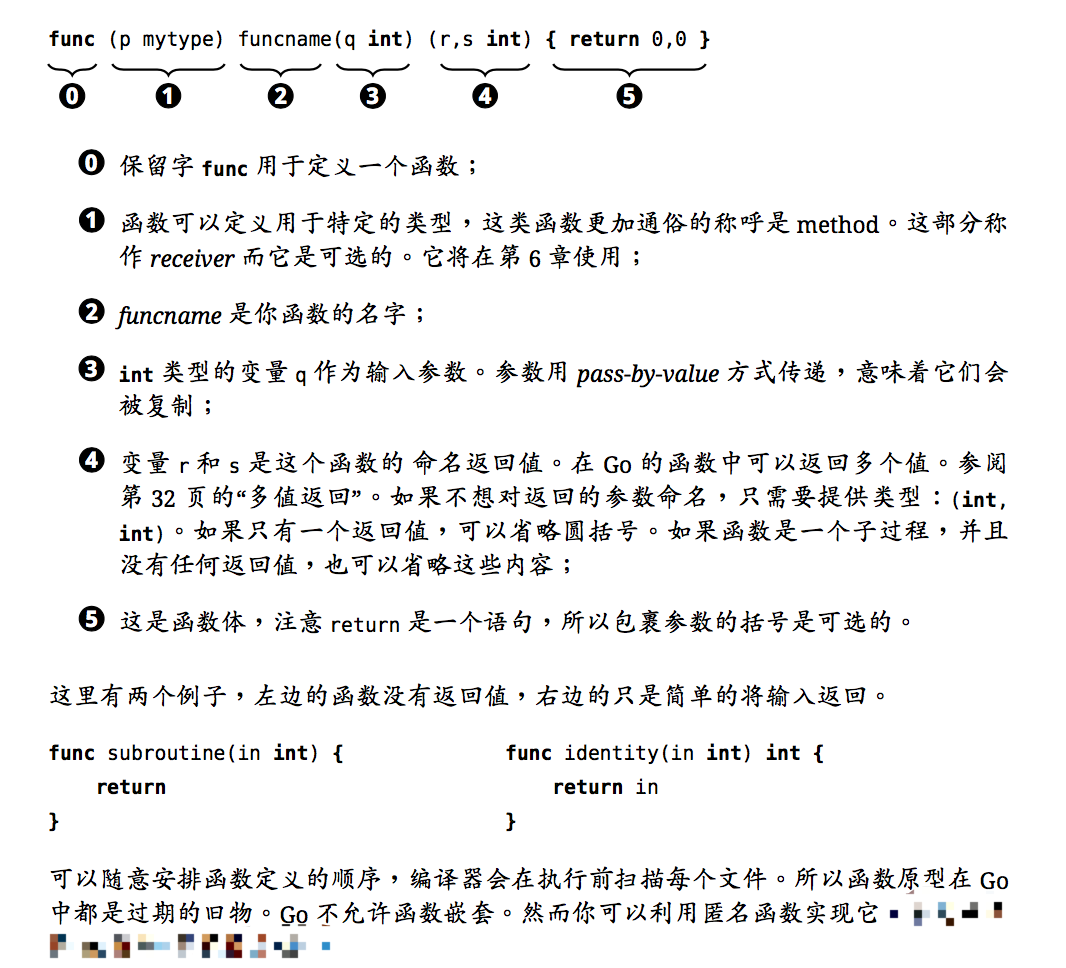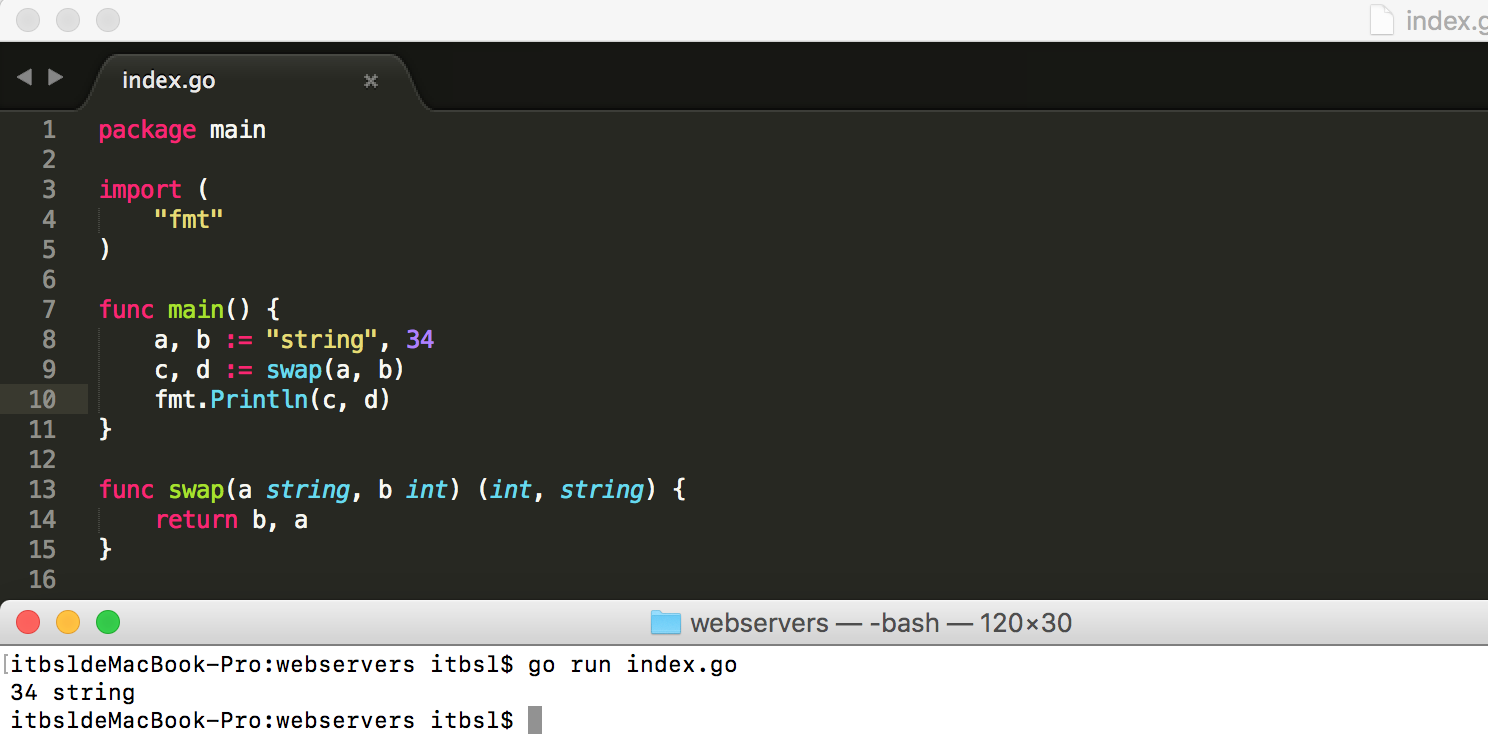设为首页 加入收藏
TOP
Go语言函数(一)
2018-10-26 14:09:17 】 浏览:156
 Tags：语言 函数

## 函数定义

Go语言函数定义格式如下:

``````func function_name ([parameter list]) [return_types] {
//函数体
}``````

• func：函数由 func 开始声明
• function_name：函数名称，函数名和参数列表一起构成了函数签名。
• parameter list：参数列表，参数就像一个占位符，当函数被调用时，你可以将值传递给参数，这个值被称为实际参数。参数列表指定的是参数类型、顺序、及参数个数。参数是可选的，也就是说函数也可以不包含参数。
• return_types：返回类型，函数返回一列值。return_types 是该列值的数据类型。有些功能不需要返回值，这种情况下 return_types 不是必须的。
• 函数体：函数定义的代码集合。``````/* 函数返回两个数的最大值 */
func max(num1, num2 int) int {
/* 声明局部变量 */
var result int

if (num1 > num2) {
result = num1
} else {
result = num2
}
return result
}``````## 函数返回多个值

Go函数可以返回多个值，例如:

``````package main

import "fmt"

func swap(x, y string) (string, string) {
return y, x
}

func main() {
a, b := swap("Mahesh", "Kumar")
fmt.Println(a, b)
}``````

## 函数参数

### Go 语言函数值传递

``````/* 定义相互交换值的函数 */
func swap(x, y int) int {
var temp int

temp = x /* 保存 x 的值 */
x = y    /* 将 y 值赋给 x */
y = temp /* 将 temp 值赋给 y*/

return temp;
}``````

``````package main

import "fmt"

func main() {
/* 定义局部变量 */
var a int = 100
var b int = 200

fmt.Printf("交换前 a 的值为 : %d\n", a )
fmt.Printf("交换前 b 的值为 : %d\n", b )

/* 通过调用函数来交换值 */
swap(a, b)

fmt.Printf("交换后 a 的值 : %d\n", a )
fmt.Printf("交换后 b 的值 : %d\n", b )
}

/* 定义相互交换值的函数 */
func swap(x, y int) int {
var temp int

temp = x /* 保存 x 的值 */
x = y    /* 将 y 值赋给 x */
y = temp /* 将 temp 值赋给 y*/

return temp;
}``````

``````交换前 a 的值为 : 100

### Go语言函数引用传递

``````/* 定义交换值函数*/
func swap(x *int, y *int) {
var temp int
temp = *x    /* 保持 x 地址上的值 */
*x = *y      /* 将 y 值赋给 x */
*y = temp    /* 将 temp 值赋给 y */
}``````

``````package main

import "fmt"

func main() {
/* 定义局部变量 */
var a int = 100
var b int= 200

fmt.Printf("交换前，a 的值 : %d\n", a )
fmt.Printf("交换前，b 的值 : %d\n", b )

/* 调用 swap() 函数
* &a 指向 a 指针，a 变量的地址
* &b 指向 b 指针，b 变量的地址
*/
swap(&a, &b)

fmt.Printf("交换后，a 的值 : %d\n", a )
fmt.Printf("交换后，b 的值 : %d\n", b )
}

func swap(x *int, y *int) {
var temp int
temp = *x    /* 保存 x 地址上的值 */
*x = *y      /* 将 y 值赋给 x */
*y = temp    /* 将 temp 值赋给 y */
}``````

``````交换前，a 的值 : 100

## 函数用法

### 函数作为值

Go 语言可以很灵活的创建函数，并作为值使用。以下实例中我们在定义的函数中初始化一个变量，该函数仅仅是为了使用内置函数 math.sqrt() ，实例为：

``````package main

import (
"fmt"
"math"
)

func main(){
/* 声明函数变量 */
getSquareRoot := func(x float64) float64 {
return math.Sqrt(x)
}

/* 使用函数 */
fmt.Println(getSquareRoot(9))

}``````

### 匿名函数

``````func main() {
res := func(n1 int, n2 int) int {
return n1 + n2
} 编程开发网 ``````

】【打印繁体】【投稿】【收藏】 【推荐】【举报】【评论】 【关闭】 【返回顶部array(4) { ["type"]=> int(8) ["message"]=> string(24) "Undefined variable: jobs" ["file"]=> string(32) "/mnt/wp/cppentry/do/bencandy.php" ["line"]=> int(214) }